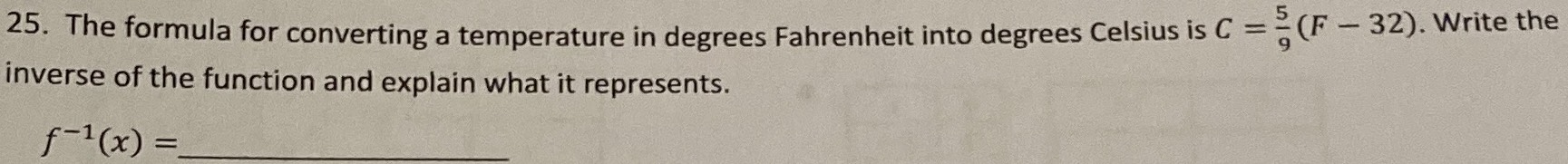### ¿Todavía tienes preguntas de matemáticas?

Pregunte a nuestros tutores expertos
Algebra
Pregunta25. The formula for converting a temperature in degrees Fahrenheit into degrees Celsius is $$C = \frac { 5 } { 9 } ( F - 32 )$$ . Write the inverse of the function and explain what it represents.$$f ^ { - 1 } ( x ) =$$

$$\frac{9}{8}x+ 32$$